Transcripted Summary

In previous chapters we dealt with primitive variables that were only able to hold one value. In this chapter we'll learn about arrays.

What are arrays?

Arrays are special objects or containers which can hold multiple values.

So, here's a typical variable is declared as an integer and can hold a single integer value.

``````int age = 1;
``````

But what if you need something to hold multiple values because collectively they are unit, like for example, a lottery ticket?

Let's say a lottery ticket is comprised of 6 integer numbers. Well, we can use an array for this.

This is the declaration for an array.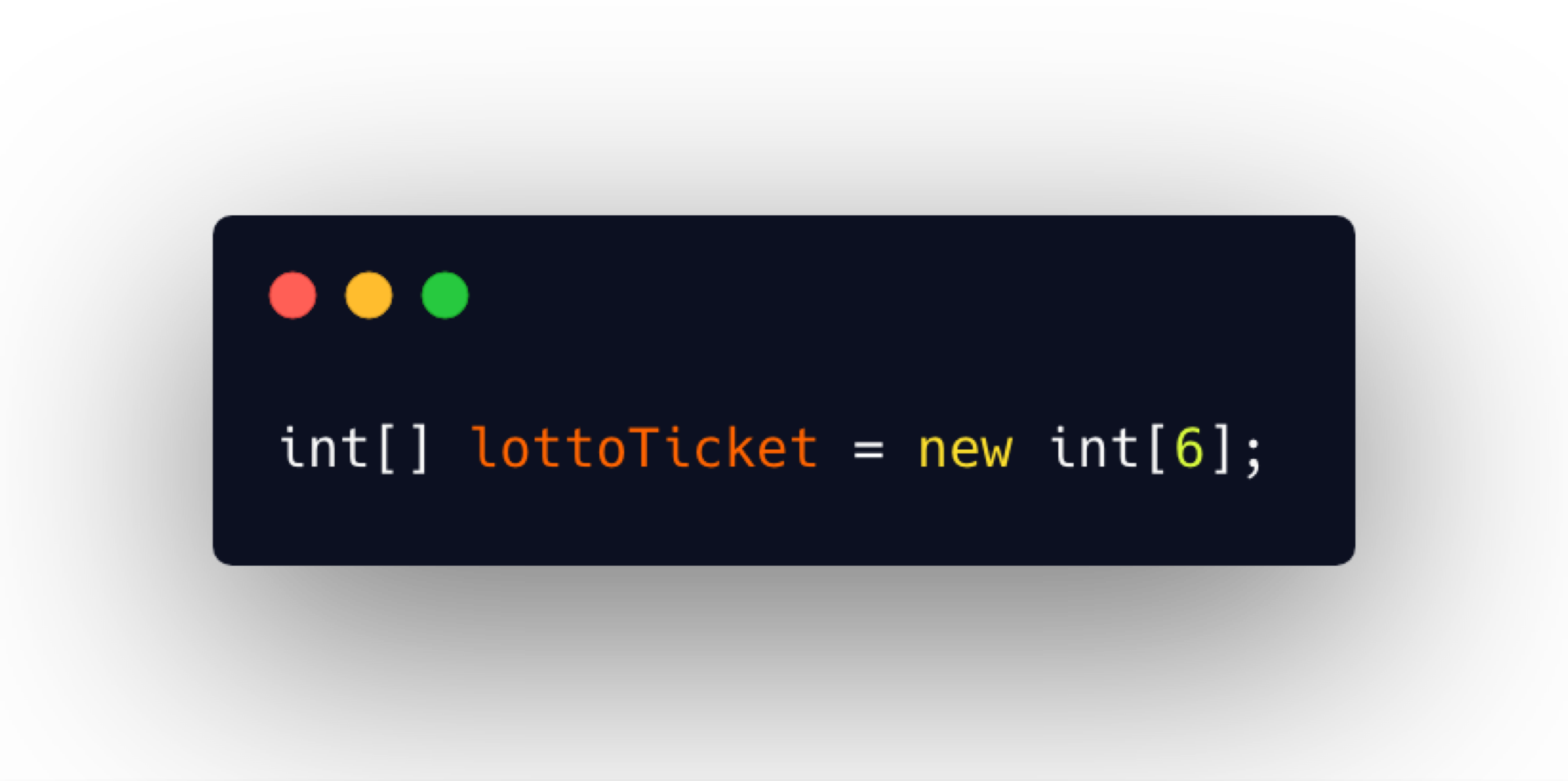Let's examine…

• `int` - the first part is the data type that the array holds and while the array is capable of holding multiple values, all values must be of the same data type

• `[]` - the square brackets indicate that this is not just an `int` data type, but this will be an array of “ints”. The bracket can appear after the data type or after the variable name itself

• `lottoTicket` - speaking of name, that's the next part of the declaration. We name arrays just as we would any other variable

• `=` - the name is followed by an equal sign

• `new` - and then we use the `new` keyword

• `int` - followed by the data type again

• ` - followed by a number inside of the brackets. This number inside of the brackets represents the length of the array, meaning how many values can this array store. Once the length is declared, it's fixed — meaning it cannot be lengthened to hold more values.

If I were to draw a picture of what an array looks like, it will resemble something like this.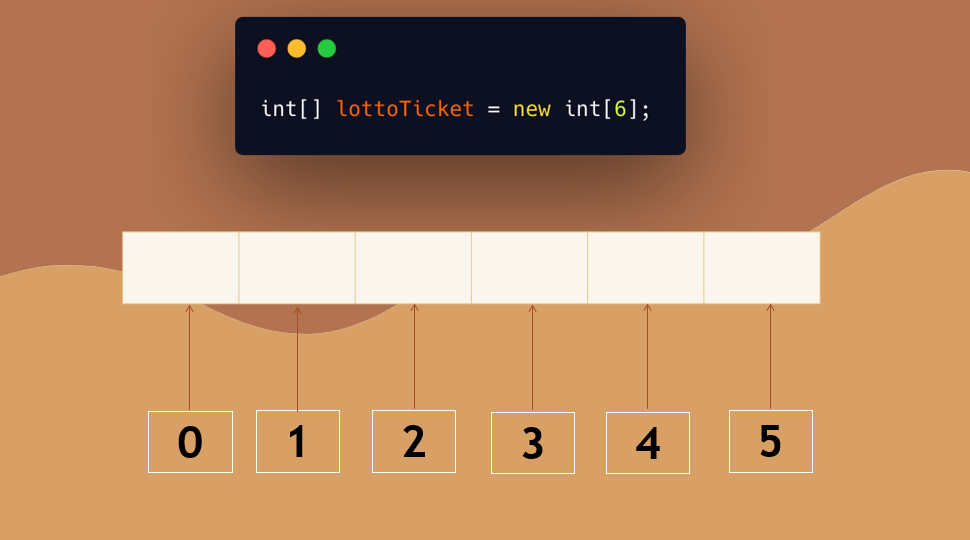There are 6 slots here because that’s the length we specified; and each of them are able to hold a single value.

These numbers at the bottom represent the indices of each space within the array. It's like an address. Each index points to one of the slots. So, if we were to have this lotto ticket array that holds six numbers, each of those numbers would be an element inside of the array.

Notice that the indices begin with 0; and index 0 represents the first element of the array.

Let's see how we add actual values to this array.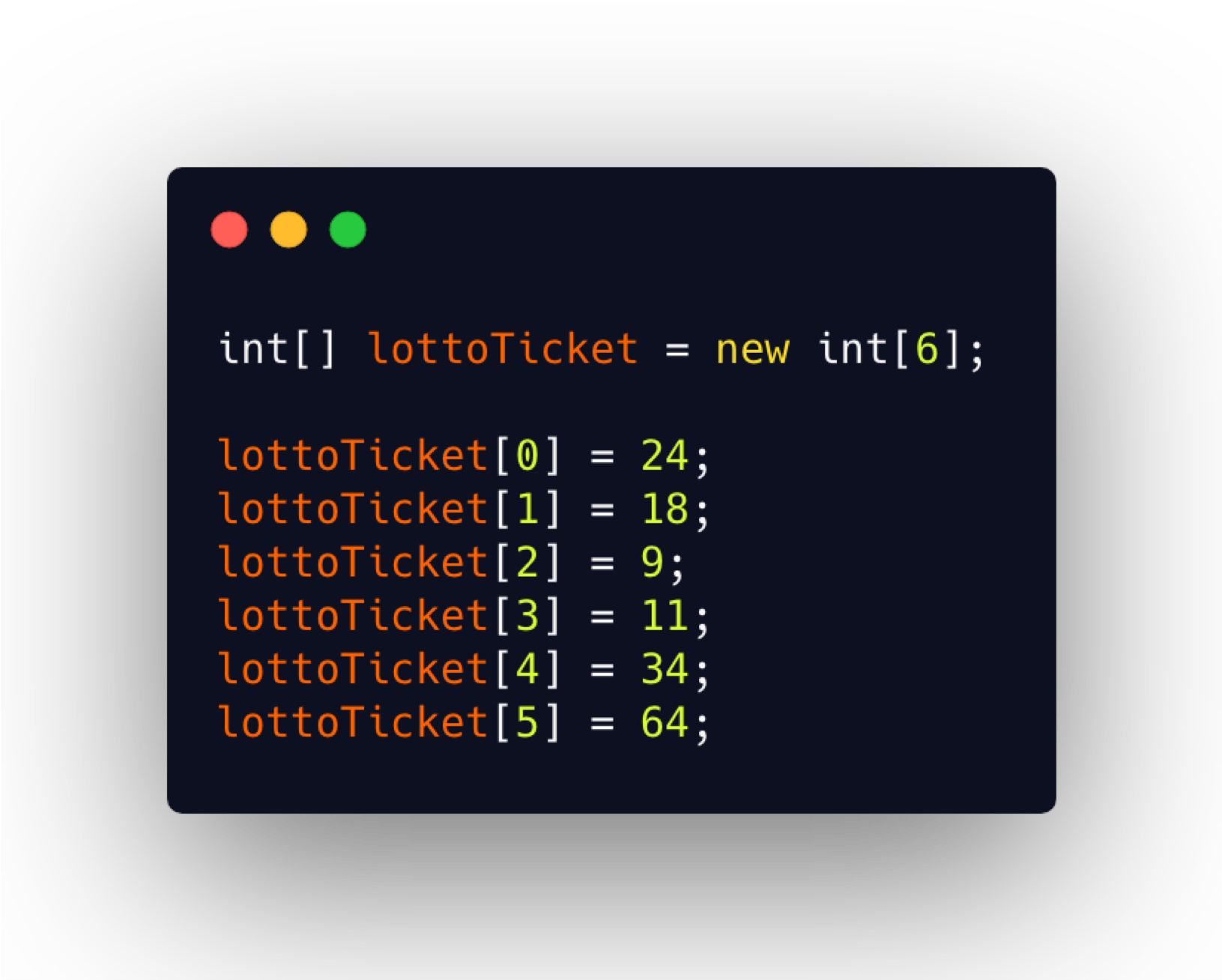Each element in an array works as an individual variable. It can be read or set by accessing its index. We can assign values to an element of the array by specifying the elements index.

• For example, we assigned 24 as the first element of the array by indicating the array's name followed by the index.
• The second element of the array will be 18; and so on.

Those values will then be stored as such: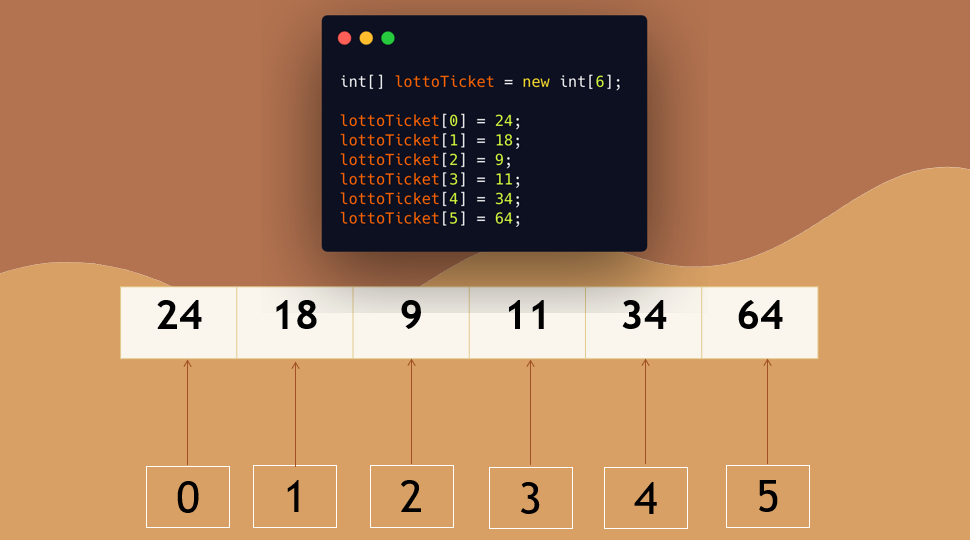And they become elements of the array.

Alternatively, if you know all values of the array's elements, you can use curly braces as a shortcut to initialize the array.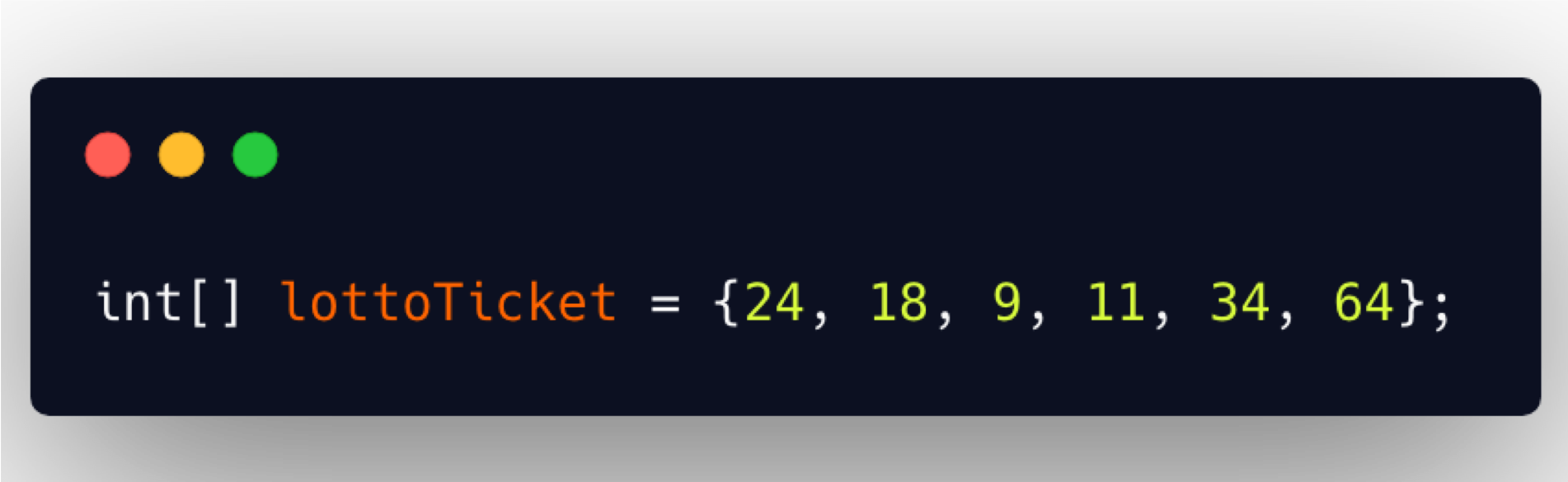This will create the new array with the length of however many values are specified within the curly braces; and they're separated with a comma. And then this will assign those values to those appropriate elements.

To read the value of an element, you simply use the array's name and the index of the element that you like.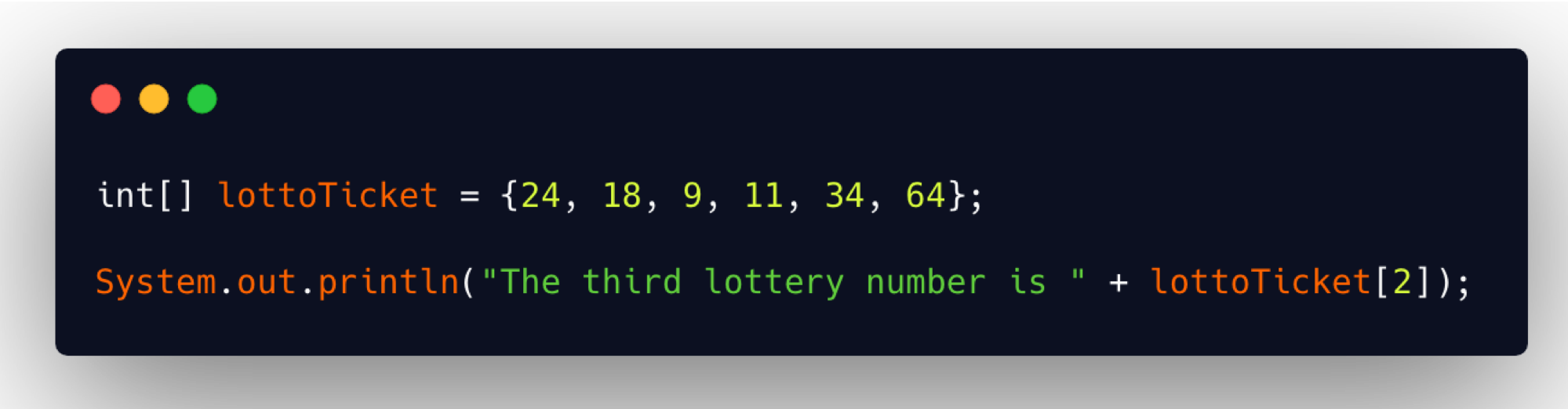In this example, we are reading out the 3rd lottery number by using the index of 2.

Let's look at an example.We're going to create a Lottery Quick Pick application that will generate a lottery ticket with 6 random numbers, between 1 and 69. I'm going to show you how to write this program, but if any one of you uses this and wins the lottery, I do expect my cut. Okay? 😉

Let's get started.

We have a new class here `LotteryTicket` in package `chapter7`:

``````package chapter7;

public class LotteryTicket {

public static void main(String[] args){

}
}
``````

I'm going to create a new method that's going to generate the lottery numbers.

Let's go ahead and make this. We'll make it `static` and it's going to `return` the lottery ticket as an array.

We specify the return data type as an array by simply specifying that data type with the brackets.

``````public static int[] generateNumbers(){

}
``````

So inside of here, we want to create a new array and let's call it `ticket`, and we'll give it a length of 6.

``````public static int[] generateNumbers(){
int[] ticket = new int;
}
``````

Now, at this point, this 6 is a magic number — who knows why this is 6?

So, we can create a variable so that this is not a magic number and we're going to call this “LENGTH” and assign it 6.

``````public class LotteryTicket {

private static int LENGTH = 6;
``````

Notice I wrote this variable name in all caps.

This is a different type of variable. I'm indicating that this is going to be a constant.

What is a constant variable?

A constant is a field within your class whose value does not change.

So, what I'm saying here is the length is always going to be 6 and that nothing within this program should update `LENGTH` to be some other number. To make sure that doesn't happen, we can add the keyword `final` here to say “this is final”.

``````private static final int LENGTH = 6;
``````

And it's in all caps because that is the convention for a constant. So, if you're going to make a constant field, you want to name it in all caps so that when people see it within the program, they know that this is a constant.

We'll go back to the `generateNumbers()` method and update this to use the constant.

``````public static int[] generateNumbers(){
int[] ticket = new int[LENGTH];
}
``````

The next thing I want to do is to randomly generate numbers to assign on the ticket; I don't want to hard-code these numbers. In order to generate random numbers, we're going to use this class called `Random`.

The Random class

This class allows us to generate random values of various data types.

There's an option to generate random integers. In fact, if you did one of the earlier optional exercises, you use the random generator for your dice game.

``````public static int[] generateNumbers(){

int[] ticket = new int[LENGTH];
Random random = new Random();
}
``````

Now we want to assign the values.

I can generate a random number and then I can assign it to one of the elements in the ticket array. However, there are 6 numbers here, which means I'm going to repeat those steps six different times. And remember if we're going to repeat something, then it's best to do it inside of a loop.

What we're going to do is create a `for` loop.

Because we know how many times we want to do it, a count-controlled loop is our best option.

``````public static int[] generateNumbers(){

int[] ticket = new int[LENGTH];
Random random = new Random();

for(int i=0; i< LENGTH; i++){

}
}
``````

Since `i` begins with 0 and array indices begin with 0, this works really nicely.

We can assign a random value to the ith element of the array. In the 1st round, this will be zero, the 2nd iteration would be 1. And so, this will increase on its own and we are going to assign it a random number.

And we see that `nextInt` is an overloaded method.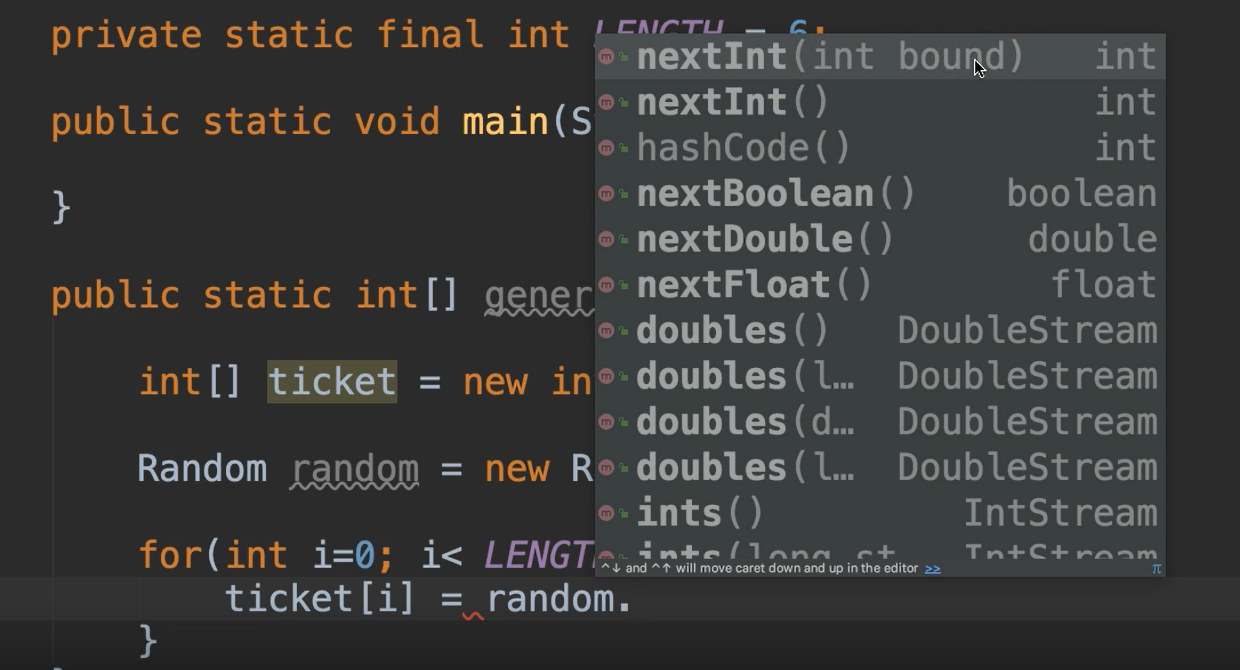There's one that takes nothing and there's one that has a bound, which means that we can say create a number between 0 and this bound.

So, we'll use the one with the bound, since our numbers have to be between 1 and 69.

``````public static int[] generateNumbers(){

int[] ticket = new int[LENGTH];
Random random = new Random();

for(int i=0; i< LENGTH; i++){
ticket[i] = random.nextInt(69)+1;
}
}
``````

The bound itself is exclusive, meaning it won't include the number that is actually set as the bound.

So, if we said “69” here, then this will create a number between 0 and 68. Because we want 1 and 69 we would need to say, “go ahead and generate whatever you're going to generate.” And then we'll add 1 to it so that we'll make sure that it's always between 1 and 69.

Now again, we have 69 here as a magic number — so I'm going to also make another constant and we'll call this `MAX_TICKET_NUMBER`.

``````public class LotteryTicket {

private static int LENGTH = 6;
private static final int MAX_TICKET_NUMBER = 69;
``````

And update this line that generates the random number to use that constant.

``````public static int[] generateNumbers(){

int[] ticket = new int[LENGTH];
Random random = new Random();

for(int i=0; i< LENGTH; i++){
ticket[i] = randomNumber = random.nextInt(MAX_TICKET_NUMBER) + 1;
}
}
``````

Okay, so that's the entire loop.

This will execute 6 times and we'll have all of those numbers populated within this ticket array.

Then we can just return the ticket: `return ticket;`

And we're good.

``````public static int[] generateNumbers(){

int[] ticket = new int[LENGTH];
Random random = new Random();

for(int i=0; i< LENGTH; i++){
ticket[i] = randomNumber = random.nextInt(MAX_TICKET_NUMBER) + 1;
}

return ticket;
}
``````

So, in our `main` method, let's go ahead and receive that ticket.

``````public static void main(String[] args){

int[] ticket = generateNumbers();
}
``````

Then we'll want to print it out.

Let's make a new method to print out an array so that I can show you how you read from an array.

This was an example of setting elements, now I want to show you how to get them.

We'll create a new method called `printTickets`:

``````public static void printTicket(){

}
``````

And this will receive the ticket, so we'll specify an array as a parameter to a method.

``````public static void printTicket(int ticket[]){

}
``````

Again, we want to print out all of the numbers. It's best to do that with a loop as opposed to trying to print them line by line. So, we use the `for` loop again.

This time we can just print those numbers out. I'm not going to use the `println`, like we usually do. I'm just going to use this `print` method instead. `println` is for “print line” — meaning there will be a line return — so, each number will print on a separate line.

Instead of that, I want all of the numbers to print on the same line — I can do that using just the `print` statement.

``````public static void printTicket(int ticket[]){
for(int i=0; i<LENGTH; i++){
System.out.print(ticket[i] + " | ");
}
}
``````

And inside of here, I'm just going to access the array element using whichever index we're on. And I'm going to go ahead and add a space so that all the numbers aren't jumbled together. And for good measure, let's add a little pipe delimiter as well.

In our main method, let's make a call to `printTicket()`.

``````public static void main(String[] args){

int[] ticket = generateNumbers();
printTicket(ticket);
}
``````

And let's run it.

### # LotteryTicket.java

``````package chapter7;

import java.util.Random;

public class LotteryTicket {

private static final int LENGTH = 6;
private static final int MAX_TICKET_NUMBER = 69;

public static void main(String[] args){

int[] ticket = generateNumbers();
printTicket(ticket);
}

public static int[] generateNumbers(){

int[] ticket = new int[LENGTH];
Random random = new Random();

for(int i=0; i< LENGTH; i++){
ticket[i] = random.nextInt(MAX_TICKET_NUMBER) + 1;
}

return ticket;
}

public static void printTicket(int ticket[]){
for(int i=0; i<LENGTH; i++){
System.out.print(ticket[i] + " | ");
}
}
}
``````

Output

``````10 | 26 | 47 | 59 | 69 | 38 |
``````

Beautiful.

So, we see here that it generated 6 random numbers.